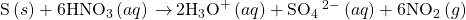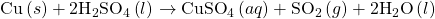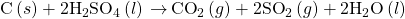# 3.11 Occurrence, Preparation, and Properties of Sulfur

Learning Objectives

By the end of this section, you will be able to:

• Describe the properties, preparation, and uses of sulfur

Sulfur exists in nature as elemental deposits as well as sulfides of iron, zinc, lead, and copper, and sulfates of sodium, calcium, barium, and magnesium. Hydrogen sulfide is often a component of natural gas and occurs in many volcanic gases, like those shown in (Figure 3.11.1). Sulfur is a constituent of many proteins and is essential for life.

The Frasch process, illustrated in (Figure 3.11.2), is important in the mining of free sulfur from enormous underground deposits in Texas and Louisiana. Superheated water (170 °C and 10 atm pressure) is forced down the outermost of three concentric pipes to the underground deposit. The hot water melts the sulfur. The innermost pipe conducts compressed air into the liquid sulfur. The air forces the liquid sulfur, mixed with air, to flow up through the outlet pipe. Transferring the mixture to large settling vats allows the solid sulfur to separate upon cooling. This sulfur is 99.5% to 99.9% pure and requires no purification for most uses.

Larger amounts of sulfur also come from hydrogen sulfide recovered during the purification of natural gas. Sulfur exists in several allotropic forms. The stable form at room temperature contains eight-membered rings, and so the true formula is S8. However, chemists commonly use S to simplify the coefficients in chemical equations; we will follow this practice in this book.

Like oxygen, which is also a member of group 16, sulfur exhibits a distinctly nonmetallic behavior. It oxidizes metals, giving a variety of binary sulfides in which sulfur exhibits a negative oxidation state (2−). Elemental sulfur oxidizes less electronegative nonmetals, and more electronegative nonmetals, such as oxygen and the halogens, will oxidize it. Other strong oxidizing agents also oxidize sulfur. For example, concentrated nitric acid oxidizes sulfur to the sulfate ion, with the concurrent formation of nitrogen(IV) oxide:The chemistry of sulfur with an oxidation state of 2− is similar to that of oxygen. Unlike oxygen, however, sulfur forms many compounds in which it exhibits positive oxidation states.

# Key Concepts and Summary

Sulfur (group 16) reacts with almost all metals and readily forms the sulfide ion, S2−, in which it has as oxidation state of 2−. Sulfur reacts with most nonmetals.

End of Chapter Exercises

(1) Explain why hydrogen sulfide is a gas at room temperature, whereas water, which has a lower molecular mass, is a liquid.

(2) Give the hybridization and oxidation state for sulfur in SO2, in SO3, and in H2SO4.

Solution

SO2, sp2 4+; SO3, sp2, 6+; H2SO4, sp3, 6+

(3) Which is the stronger acid, NaHSO3 or NaHSO4?

(4) Determine the oxidation state of sulfur in SF6, SO2F2, and KHS.

Solution

SF6: S = 6+; SO2F2: S = 6+; KHS: S = 2−

(5) Which is a stronger acid, sulfurous acid or sulfuric acid? Why?

(6) Oxygen forms double bonds in O2, but sulfur forms single bonds in S8. Why?

Solution

Sulfur is able to form double bonds only at high temperatures (substantially endothermic conditions), which is not the case for oxygen.

(7) Give the Lewis structure of each of the following:

(7a) SF4

(7b) K2SO4

(7c) SO2Cl2

(7d) H2SO3

(7e) SO3

(8) Write two balanced chemical equations in which sulfuric acid acts as an oxidizing agent.

Solution

There are many possible answers including:and(9) Explain why sulfuric acid, H2SO4, which is a covalent molecule, dissolves in water and produces a solution that contains ions.

(10) How many grams of Epsom salts (MgSO4⋅7H2O) will form from 5.0 kg of magnesium?

Solution

5.1×104 g

## Glossary

important in the mining of free sulfur from enormous underground deposits